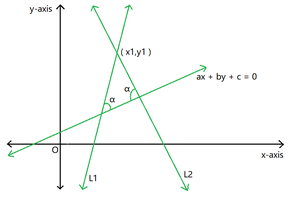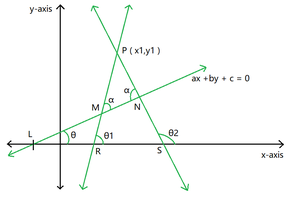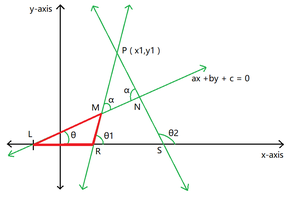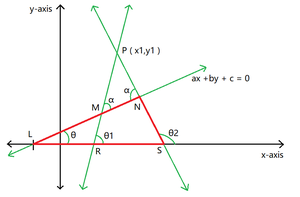# Equation of a straight line passing through a point and making a given angle with a given line

• Last Updated : 14 Jun, 2022

Given four integers a, b, c and d, representing coefficients of a straight line with equation (ax + by + c = 0), the task is to find the equations of the two straight lines passing through a given pointand making an angle α with the given straight line.Examples:

Input: a = 2, b = 3, c = -7, x1 = 4, y1 = 9, α = 30
Output: y = -0.49x +10
y = -15.51x + 71

Input: a = 3, b = -2, c = 4, x1 = 3, y1 = 4, α = 55
Output: y = 43.73x -127
y = -0.39x +5

Approach:Figure 1

• Let P (x1, y1) be the given point and line LMN (In figure 1) be the given line making an angle θ with the positive x-axis.
• Let PMR and PNS be two required lines which makes an angle (α) with the given line.
• Let these lines meet the x-axis at R and S respectively.
• Suppose line PMR and PNS make angles (θ1) and (θ2) respectively with the positive direction of the x-axis.
• Then using the slope point form of a straight line, the equation of two lines are :… (1)… (2)andare the slopes of lines PMR and PNS respectively.Figure 2

• Now consider triangle LMR:

Using the property: An exterior angle of a triangle is equal to the sum of the two opposite interior angles… (3)

• Now consider triangle LNS:Figure 3… (4)

• Now we calculate the value of (tanθ):

By formula,slope of given line• Now substitute the values of (tan(θ1)) and (tan(θ2)) from equations (3) and (4) to equations (1) and (2) to get the final equations of both the lines:

Line PMR :Line PNS :Below is the implementation of the above approach:

## C++

 `// C++ program for the above approach``#include ``using` `namespace` `std;` `// Function to find slope of given line``double` `line_slope(``double` `a, ``double` `b)``{``    ``if` `(a != 0)``        ``return` `-b / a;` `    ``// Special case when slope of``    ``// line is infinity or is``    ``// perpendicular to x-axis``    ``else``        ``return` `(-2);``}` `// Function to find equations of lines``// passing through the given point``// and making an angle with given line``void` `line_equation(``double` `a, ``double` `b,``                   ``double` `c, ``double` `x1,``                   ``double` `y1, ``double` `alfa)``{``    ``// Set the precision``    ``cout << fixed << setprecision(2);` `    ``// Store slope of given line``    ``double` `given_slope = line_slope(a, b);` `    ``// Convert degrees to radians``    ``double` `x = alfa * 3.14159 / 180;` `    ``// Special case when slope of``    ``// given line is infinity:``    ``// In this case slope of one line``    ``// will be equal to alfa``    ``// and the other line will be``    ``// equal to (180-alfa)``    ``if` `(given_slope == -2) {` `        ``// In this case slope of``        ``// required lines can't be``        ``// infinity``        ``double` `slope_1 = ``tan``(x);``        ``double` `slope_2 = ``tan``(3.14159 - x);` `        ``// g and f are the variables``        ``// of required equations``        ``int` `g = x1, f = x1;``        ``g *= (-slope_1);``        ``g += y1;` `        ``// Print first line equation``        ``if` `(g > 0)``            ``cout << ``"y = "` `<< slope_1``                 ``<< ``"x +"` `<< g << endl;``        ``if` `(g <= 0)``            ``cout << ``"y = "` `<< slope_1``                 ``<< ``"x "` `<< g << endl;` `        ``f *= (-slope_2);``        ``f += y1;` `        ``// Print second line equation``        ``if` `(f > 0) {``            ``cout << ``"y = "` `<< slope_2``                 ``<< ``"x +"` `<< f << endl;``        ``}``        ``if` `(f <= 0)``            ``cout << ``"y = "` `<< slope_2``                 ``<< ``"x "` `<< f << endl;``        ``return``;``    ``}` `    ``// Special case when slope of``    ``// required line becomes infinity``    ``if` `(1 - ``tan``(x) * given_slope == 0) {``        ``cout << ``"x = "` `<< x1 << endl;``    ``}``    ``if` `(1 + ``tan``(x) * given_slope == 0) {``        ``cout << ``"x = "` `<< x1 << endl;``    ``}` `    ``// General case``    ``double` `slope_1 = (given_slope + ``tan``(x))``                     ``/ (1 - ``tan``(x) * given_slope);``    ``double` `slope_2 = (given_slope - ``tan``(x))``                     ``/ (1 + ``tan``(x) * given_slope);` `    ``// g and f are the variables``    ``// of required equations``    ``int` `g = x1, f = x1;``    ``g *= (-slope_1);``    ``g += y1;` `    ``// Print first line equation``    ``if` `(g > 0 && 1 - ``tan``(x) * given_slope != 0)``        ``cout << ``"y = "` `<< slope_1``             ``<< ``"x +"` `<< g << endl;``    ``if` `(g <= 0 && 1 - ``tan``(x) * given_slope != 0)``        ``cout << ``"y = "` `<< slope_1``             ``<< ``"x "` `<< g << endl;``    ``f *= (-slope_2);``    ``f += y1;` `    ``// Print second line equation``    ``if` `(f > 0 && 1 + ``tan``(x) * given_slope != 0) {``        ``cout << ``"y = "` `<< slope_2``             ``<< ``"x +"` `<< f << endl;``    ``}``    ``if` `(f <= 0 && 1 + ``tan``(x) * given_slope != 0)``        ``cout << ``"y = "` `<< slope_2``             ``<< ``"x "` `<< f << endl;``}` `// Driver Code``int` `main()``{``    ``// Given Input``    ``double` `a = 2, b = 3, c = -7;``    ``double` `x1 = 4, y1 = 9;``    ``double` `alfa = 30;` `    ``// Function Call``    ``line_equation(a, b, c, x1, y1, alfa);` `    ``return` `0;``}`

## Java

 `// Java program for the above approach``import` `java.io.*;` `class` `GFG{``  ` `// Function to find slope of given line``static` `double` `line_slope(``double` `a, ``double` `b)``{``    ``if` `(a != ``0``)``        ``return` `-b / a;` `    ``// Special case when slope of``    ``// line is infinity or is``    ``// perpendicular to x-axis``    ``else``        ``return` `(-``2``);``}` `// Function to find equations of lines``// passing through the given point``// and making an angle with given line``static` `void` `line_equation(``double` `a, ``double` `b,``                          ``double` `c, ``double` `x1,``                          ``double` `y1, ``double` `alfa)``{``    ` `    ``// Store slope of given line``    ``double` `given_slope = line_slope(a, b);` `    ``// Convert degrees to radians``    ``double` `x = alfa * ``3.14159` `/ ``180``;` `    ``// Special case when slope of``    ``// given line is infinity:``    ``// In this case slope of one line``    ``// will be equal to alfa``    ``// and the other line will be``    ``// equal to (180-alfa)``    ``if` `(given_slope == -``2``)``    ``{``        ` `        ``// In this case slope of``        ``// required lines can't be``        ``// infinity``        ``double` `slope_1 = Math.tan(x);``        ``double` `slope_2 = Math.tan(``3.14159` `- x);` `        ``// g and f are the variables``        ``// of required equations``        ``int` `g = (``int``)x1, f = (``int``)x1;``        ``g *= (-slope_1);``        ``g += y1;` `        ``// Print first line equation``        ``if` `(g > ``0``)``            ``System.out.println(``"y = "` `+``            ``(Math.round(slope_1 * ``100.0``) / ``100.0``) +``          ``"x +"` `+ (Math.round(g * ``100.0``) / ``100.0``));``        ``if` `(g <= ``0``)``             ``System.out.println(``"y = "` `+``             ``(Math.round(slope_1 * ``100.0``) / ``100.0``) +``            ``"x "` `+ (Math.round(g * ``100.0``) / ``100.0``));` `        ``f *= (-slope_2);``        ``f += y1;` `        ``// Print second line equation``        ``if` `(f > ``0``)``        ``{``             ``System.out.println(``"y = "` `+``             ``(Math.round(slope_2 * ``100.0``) / ``100.0``) +``           ``"x +"` `+ (Math.round(f * ``100.0``) / ``100.0``));``        ``}``        ``if` `(f <= ``0``)``             ``System.out.println(``"y = "` `+``             ``(Math.round(slope_1 * ``100.0``) / ``100.0``) +``            ``"x "` `+ (Math.round(g * ``100.0``) / ``100.0``));``        ``return``;``    ``}` `    ``// Special case when slope of``    ``// required line becomes infinity``    ``if` `(``1` `- Math.tan(x) * given_slope == ``0``)``    ``{``         ``System.out.println(``"x = "` `+``         ``(Math.round(x1 * ``100.0``) / ``100.0``));``    ``}``    ``if` `(``1` `+ Math.tan(x) * given_slope == ``0``)``    ``{``        ``System.out.println(``"x = "` `+``        ``(Math.round(x1 * ``100.0``) / ``100.0``));``    ``}` `    ``// General case``    ``double` `slope_1 = (given_slope + Math.tan(x)) /``                 ``(``1` `- Math.tan(x) * given_slope);``    ``double` `slope_2 = (given_slope - Math.tan(x)) /``                 ``(``1` `+ Math.tan(x) * given_slope);` `    ``// g and f are the variables``    ``// of required equations``    ``int` `g = (``int``)x1, f = (``int``)x1;``    ``g *= (-slope_1);``    ``g += y1;` `    ``// Print first line equation``    ``if` `(g > ``0` `&& ``1` `- Math.tan(x) * given_slope != ``0``)``          ``System.out.println(``"y = "` `+``          ``(Math.round(slope_1 * ``100.0``) / ``100.0``) +``      ``"x +"` `+ (Math.round(g * ``100.0``) / ``100.0``));``    ``if` `(g <= ``0` `&& ``1` `- Math.tan(x) * given_slope != ``0``)``       ``System.out.println(``"y = "` `+``       ``(Math.round(slope_1 * ``100.0``) / ``100.0``) +``      ``"x "` `+ (Math.round(g * ``100.0``) / ``100.0``));``      ` `    ``f *= (-slope_2);``    ``f += y1;` `    ``// Print second line equation``    ``if` `(f > ``0` `&& ``1` `+ Math.tan(x) * given_slope != ``0``)``    ``{``        ``System.out.println(``"y = "` `+``        ``(Math.round(slope_2 * ``100.0``) / ``100.0``) +``      ``"x +"` `+ (Math.round(f * ``100.0``) / ``100.0``));``    ``}``    ``if` `(f <= ``0` `&& ``1` `+ Math.tan(x) * given_slope != ``0``)``        ``System.out.println(``"y = "` `+``        ``(Math.round(slope_2 * ``100.0``) / ``100.0``) +``      ``"x +"` `+ (Math.round(f * ``100.0``) / ``100.0``));``}` `// Driver Code``public` `static` `void` `main (String[] args)``{``    ` `    ``// Given Input``    ``double` `a = ``2``, b = ``3``, c = -``7``;``    ``double` `x1 = ``4``, y1 = ``9``;``    ``double` `alfa = ``30``;``    ` `    ``// Function Call``    ``line_equation(a, b, c, x1, y1, alfa);``}``}` `// This code is contributed by Dharanendra L V.`

## Python3

 `# Python3 program for the above approach``import` `math` `# Function to find slope of given line``def` `line_slope(a, b):` `    ``if` `(a !``=` `0``):``        ``return` `-``b ``/` `a` `    ``# Special case when slope of``    ``# line is infinity or is``    ``# perpendicular to x-axis``    ``else``:``        ``return` `(``-``2``)` `# Function to find equations of lines``# passing through the given point``# and making an angle with given line``def` `line_equation(a, b, c, x1, y1, alfa):``    ` `    ``# Store slope of given line``    ``given_slope ``=` `line_slope(a, b)` `    ``# Convert degrees to radians``    ``x ``=` `alfa ``*` `3.14159` `/` `180` `    ``# Special case when slope of``    ``# given line is infinity:``    ``# In this case slope of one line``    ``# will be equal to alfa``    ``# and the other line will be``    ``# equal to (180-alfa)``    ``if` `(given_slope ``=``=` `-``2``):` `        ``# In this case slope of``        ``# required lines can't be``        ``# infinity``        ``slope_1 ``=` `math.tan(x)``        ``slope_2 ``=` `math.tan(``3.14159` `-` `x)` `        ``# g and f are the variables``        ``# of required equations``        ``g ``=` `x1, f ``=` `x1``        ``g ``*``=` `(``-``slope_1)``        ``g ``+``=` `y1` `        ``# Print first line equation``        ``if` `(g > ``0``):``            ``print``(``"y = "``, ``round``(slope_1, ``2``),``                  ``"x +"` `, ``round``(g));``        ``if` `(g <``=` `0``):``            ``print``(``"y = "``, ``round``(slope_1, ``2``),``                  ``"x "``, ``round``(g))` `        ``f ``*``=` `(``-``slope_2)``        ``f ``+``=` `y1` `        ``# Print second line equation``        ``if` `(f > ``0``):``            ``print``(``"y = "``, ``round``(slope_2, ``2``),``                  ``"x +"``, ``round``(f))``        ` `        ``if` `(f <``=` `0``):``            ``print``(``"y = "` `, ``round``(slope_2, ``2``),``                  ``"x "` `, ``round``(f))``        ``return``    ` `    ``# Special case when slope of``    ``# required line becomes infinity``    ``if` `(``1` `-` `math.tan(x) ``*` `given_slope ``=``=` `0``):``        ``print``(``"x ="``, x1)``    ` `    ``if` `(``1` `+` `math.tan(x) ``*` `given_slope ``=``=` `0``):``        ``print``(``"x ="``, x1)``    ` `    ``# General case``    ``slope_1 ``=` `((given_slope ``+` `math.tan(x)) ``/``           ``(``1` `-` `math.tan(x) ``*` `given_slope))``    ``slope_2 ``=` `((given_slope ``-` `math.tan(x)) ``/``           ``(``1` `+` `math.tan(x) ``*` `given_slope))` `    ``# g and f are the variables``    ``# of required equations``    ``g ``=` `x1``    ``f ``=` `x1``    ``g ``*``=` `(``-``slope_1)``    ``g ``+``=` `y1` `    ``# Print first line equation``    ``if` `(g > ``0` `and` `1` `-` `math.tan(x) ``*` `given_slope !``=` `0``):``        ``print``(``"y = "``, ``round``(slope_1, ``2``),``              ``"x +"``, ``round``(g))``    ``if` `(g <``=` `0` `and` `1` `-` `math.tan(x) ``*` `given_slope !``=` `0``):``        ``print``(``"y = "``, ``round``(slope_1, ``2``),``              ``"x "``, ``round``(g))``              ` `    ``f ``*``=` `(``-``slope_2)``    ``f ``+``=` `y1` `    ``# Print second line equation``    ``if` `(f > ``0` `and` `1` `+` `math.tan(x) ``*` `given_slope !``=` `0``):``        ``print``(``"y = "``, ``round``(slope_2, ``2``),``              ``"x +"``, ``round``(f))``    ` `    ``if` `(f <``=` `0` `and` `1` `+` `math.tan(x) ``*` `given_slope !``=` `0``):``        ``print``(``"y = "``, ``round``(slope_2, ``2``),``              ``"x "` `, ``round``(f))``              ` `# Driver Code``if` `__name__ ``=``=` `"__main__"``:` `    ``# Given Input``    ``a ``=` `2``    ``b ``=` `3``    ``c ``=` `-``7``    ``x1 ``=` `4``    ``y1 ``=` `9``    ``alfa ``=` `30``    ` `    ``# Function Call``    ``line_equation(a, b, c, x1, y1, alfa)` `# This code is contributed by ukasp`

## C#

 `// C# program for the above approach`  `using` `System;``using` `System.Collections.Generic;``public` `class` `GFG{``  ` `// Function to find slope of given line``static` `double` `line_slope(``double` `a, ``double` `b)``{``    ``if` `(a != 0)``        ``return` `-b / a;` `    ``// Special case when slope of``    ``// line is infinity or is``    ``// perpendicular to x-axis``    ``else``        ``return` `(-2);``}` `// Function to find equations of lines``// passing through the given point``// and making an angle with given line``static` `void` `line_equation(``double` `a, ``double` `b,``                          ``double` `c, ``double` `x1,``                          ``double` `y1, ``double` `alfa)``{``    ` `    ``// Store slope of given line``    ``double` `given_slope = line_slope(a, b);` `    ``// Convert degrees to radians``    ``double` `x = alfa * 3.14159 / 180;``    ``double` `slope_1,slope_2;``    ``double` `g,f;``    ``// Special case when slope of``    ``// given line is infinity:``    ``// In this case slope of one line``    ``// will be equal to alfa``    ``// and the other line will be``    ``// equal to (180-alfa)``    ``if` `(given_slope == -2)``    ``{``        ` `        ``// In this case slope of``        ``// required lines can't be``        ``// infinity``        ``slope_1 = Math.Tan(x);``        ``slope_2 = Math.Tan(3.14159 - x);` `        ``// g and f are the variables``        ``// of required equations``        ``g = (``int``)x1;``        ``f = (``int``)x1;``        ``g *= (-slope_1);``        ``g += y1;` `        ``// Print first line equation``        ``if` `(g > 0)``            ``Console.WriteLine(``"y = "` `+``            ``(Math.Round(slope_1 * 100.0) / 100.0) +``          ``"x +"` `+ (Math.Round((``int``)g * 100.0) / 100.0));``        ``if` `(g <= 0)``             ``Console.WriteLine(``"y = "` `+``             ``(Math.Round(slope_1 * 100.0) / 100.0) +``            ``"x "` `+ (Math.Round((``int``)g * 100.0) / 100.0));` `        ``f *= (-slope_2);``        ``f += y1;` `        ``// Print second line equation``        ``if` `(f > 0)``        ``{``             ``Console.WriteLine(``"y = "` `+``             ``(Math.Round(slope_2 * 100.0) / 100.0) +``           ``"x +"` `+ (Math.Round((``int``)f * 100.0) / 100.0));``        ``}``        ``if` `(f <= 0)``             ``Console.WriteLine(``"y = "` `+``             ``(Math.Round(slope_1 * 100.0) / 100.0) +``            ``"x "` `+ (Math.Round((``int``)g * 100.0) / 100.0));``        ``return``;``    ``}` `    ``// Special case when slope of``    ``// required line becomes infinity``    ``if` `(1 - Math.Tan(x) * given_slope == 0)``    ``{``         ``Console.WriteLine(``"x = "` `+``         ``(Math.Round(x1 * 100.0) / 100.0));``    ``}``    ``if` `(1 + Math.Tan(x) * given_slope == 0)``    ``{``        ``Console.WriteLine(``"x = "` `+``        ``(Math.Round(x1 * 100.0) / 100.0));``    ``}` `    ``// General case``    ``slope_1 = (given_slope + Math.Tan(x)) /``                 ``(1 - Math.Tan(x) * given_slope);``    ``slope_2 = (given_slope - Math.Tan(x)) /``                 ``(1 + Math.Tan(x) * given_slope);` `    ``// g and f are the variables``    ``// of required equations``    ``g = (``int``)x1;``    ``f = (``int``)x1;``    ``g *= (-slope_1);``    ``g += y1;` `    ``// Print first line equation``    ``if` `(g > 0 && 1 - Math.Tan(x) * given_slope != 0)``          ``Console.WriteLine(``"y = "` `+``          ``(Math.Round(slope_1 * 100.0) / 100.0) +``      ``"x +"` `+ (Math.Round((``int``)g * 100.0) / 100.0));``    ``if` `(g <= 0 && 1 - Math.Tan(x) * given_slope != 0)``       ``Console.WriteLine(``"y = "` `+``       ``(Math.Round(slope_1 * 100.0) / 100.0) +``      ``"x "` `+ (Math.Round((``int``)g * 100.0) / 100.0));``      ` `    ``f *= (-slope_2);``    ``f += y1;` `    ``// Print second line equation``    ``if` `(f > 0 && 1 + Math.Tan(x) * given_slope != 0)``    ``{``        ``Console.WriteLine(``"y = "` `+``        ``(Math.Round(slope_2 * 100.0) / 100.0) +``      ``"x +"` `+ (Math.Round((``int``)f * 100.0) / 100.0));``    ``}``    ``if` `(f <= 0 && 1 + Math.Tan(x) * given_slope != 0)``        ``Console.WriteLine(``"y = "` `+``        ``(Math.Round(slope_2 * 100.0) / 100.0) +``      ``"x +"` `+ (Math.Round((``int``)f * 100.0) / 100.0));``}` `// Driver Code``public` `static` `void` `Main(String[] args)``{``    ` `    ``// Given Input``    ``double` `a = 2, b = 3, c = -7;``    ``double` `x1 = 4, y1 = 9;``    ``double` `alfa = 30;``    ` `    ``// Function Call``    ``line_equation(a, b, c, x1, y1, alfa);``}``}` `// This code contributed by shikhasingrajput`

## Javascript

 `// JavaScript program for the above approach` `// Function to find slope of given line``function` `line_slope(a, b)``{``    ``if` `(a != 0)``        ``return` `-b / a;` `    ``// Special case when slope of``    ``// line is infinity or is``    ``// perpendicular to x-axis``    ``else``        ``return` `(-2);``}` `// Function to find equations of lines``// passing through the given point``// and making an angle with given line``function` `line_equation(a, b, c, x1, y1, alfa)``{``    ` `    ``// Store slope of given line``    ``let given_slope = line_slope(a, b);` `    ``// Convert degrees to radians``    ``let x = alfa * 3.14159 / 180;` `    ``// Special case when slope of``    ``// given line is infinity:``    ``// In this case slope of one line``    ``// will be equal to alfa``    ``// and the other line will be``    ``// equal to (180-alfa)``    ``if` `(given_slope == -2) {` `        ``// In this case slope of``        ``// required lines can't be``        ``// infinity``        ``let slope_1 = Math.tan(x);``        ``let slope_2 = Math.tan(3.14159 - x);` `        ``// g and f are the variables``        ``// of required equations``        ``let g = x1, f = x1;``        ``g = g*(-slope_1);``        ``g = g + y1;` `        ``// Print first line equation``        ``if` `(g > 0)``            ``console.log(``"y = "``, slope_1.toFixed(2), ``"x +"``, Math.floor(g));``        ``if` `(g <= 0)``            ``console.log(``"y = "``, slope_1.toFixed(2), ``"x "``, Math.floor(g));` `        ``f = f*(-slope_2);``        ``f = f+y1;` `        ``// Print second line equation``        ``if` `(f > 0) {``            ``console.log(``"y = "``, slope_2.toFixed(2), ``"x +"``, Math.floor(f));``        ``}``        ``if` `(f <= 0){``            ``console.log(``"y = "``, slope_2.toFixed(2), ``"x "``, Math.floor(f));``        ``}``        ``return``;``    ``}` `    ``// Special case when slope of``    ``// required line becomes infinity``    ``if` `(1 - Math.tan(x) * given_slope == 0) {``        ``console.log(``"x = "``, x1.toFixed(2));``    ``}``    ``if` `(1 + Math.tan(x) * given_slope == 0) {``        ``console.log(``"x = "``, x1.toFixed(2));``    ``}` `    ``// General case``    ``let slope_1 = (given_slope + Math.tan(x))``                     ``/ (1 - Math.tan(x) * given_slope);``    ``let slope_2 = (given_slope - Math.tan(x))``                     ``/ (1 + Math.tan(x) * given_slope);` `    ``// g and f are the variables``    ``// of required equations``    ``let g = x1, f = x1;``    ``g *= (-slope_1);``    ``g += y1;` `    ``// Print first line equation``    ``if` `(g > 0 && 1 - Math.tan(x) * given_slope != 0)``        ``console.log(``"y = "``, slope_1.toFixed(2), ``"x +"``, Math.floor(g));``    ``if` `(g <= 0 && 1 - tan(x) * given_slope != 0)``        ``console.log(``"y = "``, slope_1.toFixed(2), ``"x "``, Math.floor(g));``    ` `    ``f *= (-slope_2);``    ``f += y1;` `    ``// Print second line equation``    ``if` `(f > 0 && 1 + Math.tan(x) * given_slope != 0) {``        ``console.log(``"y = "``, slope_2.toFixed(2), ``"x +"``, Math.floor(f));``    ``}``    ``if` `(f <= 0 && 1 + tan(x) * given_slope != 0)``        ``console.log(``"y = "``, slope_2.toFixed(2), ``"x "``, Math.floor(f));``}` `// Driver Code` `// Given Input``let a = 2, b = 3, c = -7;``let x1 = 4, y1 = 9;``let alfa = 30;` `// Function Call``line_equation(a, b, c, x1, y1, alfa);` `// The code is contributed by Gautam goel (gautamgoel962)`

Output:

```y = -0.49x +10
y = -15.51x +71```

Time Complexity: O(1)
Auxiliary Space: O(1)

My Personal Notes arrow_drop_up